# Spiral Level Order Traversal of a Binary Tree | Set 1

Given a binary tree, write a program to print nodes of the tree in spiral order. For example, for the following tree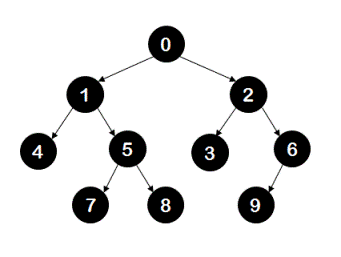output should be 0,1,2,6,3,5,4,7,8,9.

## Algorithm/Insights

Idea: The idea to traverse the given binary tree in spiral order is similar to level order traversal.

In level order traversal we make use of a single queue while in spiral order traversal we need use two stacks. First stack('stackEven') is used to traverse the nodes at even level and second stack('stackOdd') is used to traverse the nodes at odd level. Nodes at even level are stored in 'stackEven' and are pushed in it from left to right order whereas nodes at odd level are stored in 'stackOdd' and are pushed in it from right to left order.

Algorithm:
1. Push root node into the 'stackEven'. Set boolean 'evenLevel' to true. This boolean variable is used to indicate if the current level being visited is even level or odd level.

2. Now if 'evenLevel' is set to true repeat steps 2a to 2c until 'stackEven' is not empty.
2a. Pop the node from 'stackEven'. Let popped node be 'currentNode'. Print the value of 'currentNode'.
2b. If the right child of 'currentNode' is not null, push it into the 'stackOdd'.
2c. If the left child of 'currentNode' is not null, push it into the 'stackOdd'.
Notice that currentNode's right child is pushed first and then its left child is pushed.

3. If 'evenLevel' is not set to true then repeat steps 3a to 3c until 'stackOdd' is not empty.
3a. Pop the node from 'stackOdd'. Let popped node be 'currentNode'. Print the value of 'currentNode'.
3b. If the left child of 'currentNode' is not null, push it into the 'stackEven'.
3c. If the right child of 'currentNode' is not null, push it into the 'stackEven'.
Notice that currentNode's left child is pushed first and then its right child is pushed.

4. If 'evenLevel' is true set it to false and if it is false set it to true. This is because the next level visited would be odd if current level is even and vice versa.

5. Repeat steps 2-4 until both 'stackEven' and 'stackOdd' are not empty.
Implementation details could be found in function 'spiralTraversal(TreeNode root)' in the code snippet.

Example: Let's look at the state of the stacks as nodes in each level are visited for the following tree.Initially, root node is pushed into 'stackEven'.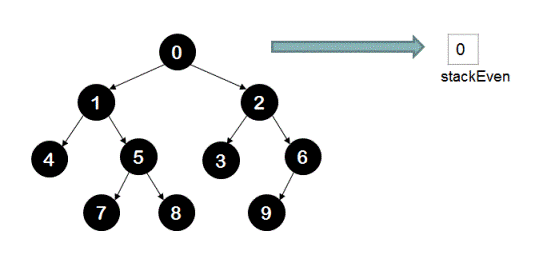Then root node is popped from 'stackEven' and its child nodes are pushed into 'stackOdd' from right to left order.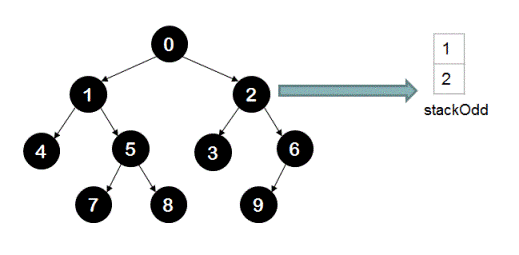All nodes from 'stackOdd' are popped and their child nodes are pushed into 'stackEven' from left to right order.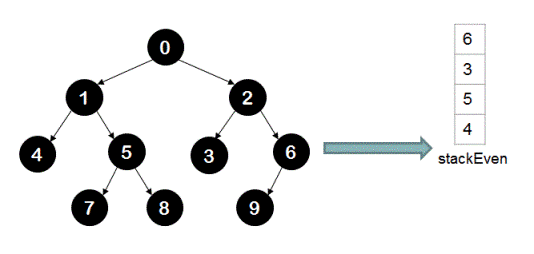All nodes from 'stackEven' are popped and their child nodes are pushed into 'stackOdd' from right to left order.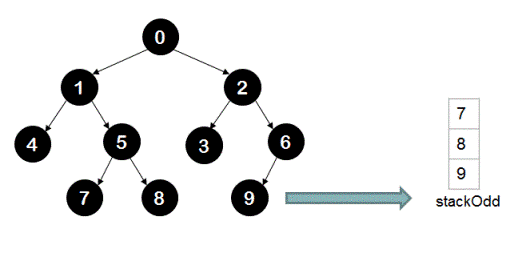Finally all nodes from 'stackOdd' are popped.

## Code Snippet

```
package com.ideserve.nilesh.questions;

/**
* <b>IDeserve <br>
* Visits nodes of a binary tree in spiral level order using stacks.
* @author Nilesh
*/

import java.util.Stack;

public class SpiralTraversal
{
private class TreeNode
{
TreeNode left;
TreeNode right;
int value;

public TreeNode(int value)
{
this.value = value;
}
}

TreeNode root;

/*
0
1              2
4      5       3     6
7   8         9
*/
public void createTree()
{
this.root = new TreeNode(0);

TreeNode n1 = new TreeNode(1);
TreeNode n2 = new TreeNode(2);
TreeNode n3 = new TreeNode(3);
TreeNode n4 = new TreeNode(4);
TreeNode n5 = new TreeNode(5);
TreeNode n6 = new TreeNode(6);
TreeNode n7 = new TreeNode(7);
TreeNode n8 = new TreeNode(8);
TreeNode n9 = new TreeNode(9);

root.left  = n1;
root.right = n2;

n1.left  =  n4;
n1.right = n5;

n2.left  = n3;
n2.right = n6;

n5.left  = n7;
n5.right = n8;

n6.left  = n9;
}

private void spiralTraversal(TreeNode root)
{
if (root == null)
{
return;
}

// for storing and printing nodes at even level
Stack<TreeNode> stackEven = new Stack();

// for storing and printing nodes at odd level
Stack<TreeNode> stackOdd = new Stack();

// root node is considered at level 0.
stackEven.push(root);

boolean evenLevel = true;

// traverse while there are nodes to visit in the current-level stack
// empty current-level stack indicates that all nodes of the tree are visited
while ((evenLevel && !stackEven.isEmpty()) || (!stackOdd.isEmpty()))
{
if (evenLevel) // if current level to be visited is even
{
// visit nodes at even level and push their children in odd level stack
while (!stackEven.isEmpty())
{
TreeNode currentNode = stackEven.pop();

System.out.print(" " + currentNode.value);

// first push the right child
if (currentNode.right != null)
{
stackOdd.push(currentNode.right);
}

// then push the left child
if (currentNode.left != null)
{
stackOdd.push(currentNode.left);
}
}
}
else // if current level to be visited is odd
{
// visit nodes at odd level and push their children in even level stack
while (!stackOdd.isEmpty())
{
TreeNode currentNode = stackOdd.pop();

System.out.print(" " + currentNode.value);

// first push the left child
if (currentNode.left != null)
{
stackEven.push(currentNode.left);
}

// then push the right child
if (currentNode.right != null)
{
stackEven.push(currentNode.right);
}
}
}

// if current level is even switch to odd and vice versa
evenLevel = !evenLevel;
System.out.println();
}
}

public void spiralTraversal()
{
spiralTraversal(this.root);
}

public static void main(String[] args)
{
SpiralTraversal solution = new SpiralTraversal();

/*
0
1              2
4      5       3     6
7   8         9
*/
solution.createTree();

solution.spiralTraversal();
}
}
```

## Order of the Algorithm

Time Complexity is O(n)
Space Complexity is O(n)